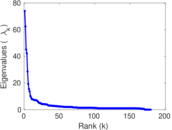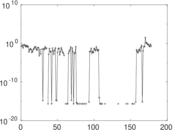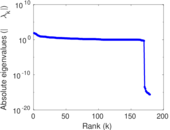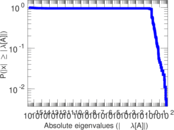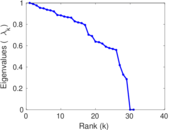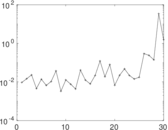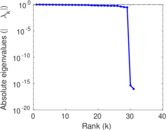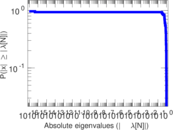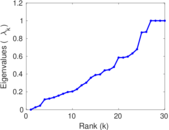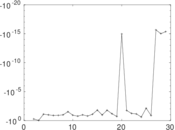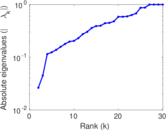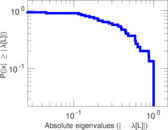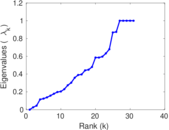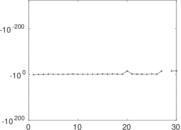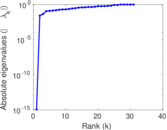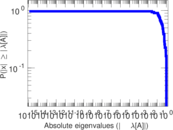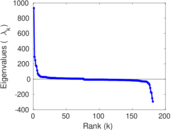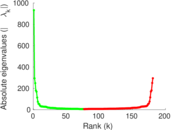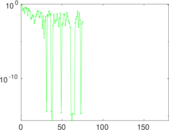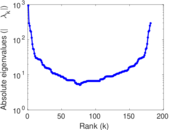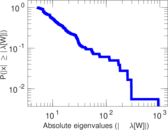# Wikibooks edits (te)

This is the bipartite edit network of the Telugu Wikibooks. It contains users and pages from the Telugu Wikibooks, connected by edit events. Each edge represents an edit. The dataset includes the timestamp of each edit.

 Code `bte` Internal name `edit-tewikibooks` Name Wikibooks edits (te) Data source http://dumps.wikimedia.org/ AvailabilityDataset is available for download Consistency checkDataset passed all tests Category Authorship network Dataset timestamp 2017-10-20 Node meaning User, article Edge meaning Edit Network formatBipartite, undirected Edge typeUnweighted, multiple edges Temporal dataEdges are annotated with timestamps

## Statistics

 Size n = 944 Left size n1 = 181 Right size n2 = 763 Volume m = 1,646 Unique edge count m̿ = 888 Wedge count s = 26,273 Claw count z = 1,172,821 Cross count x = 46,693,881 Square count q = 2,755 4-Tour count T4 = 128,952 Maximum degree dmax = 268 Maximum left degree d1max = 268 Maximum right degree d2max = 67 Average degree d = 3.487 29 Average left degree d1 = 9.093 92 Average right degree d2 = 2.157 27 Fill p = 0.006 429 98 Average edge multiplicity m̃ = 1.853 60 Size of LCC N = 275 Diameter δ = 11 50-Percentile effective diameter δ0.5 = 3.184 93 90-Percentile effective diameter δ0.9 = 7.082 71 Median distance δM = 4 Mean distance δm = 3.975 53 Gini coefficient G = 0.670 296 Balanced inequality ratio P = 0.241 798 Left balanced inequality ratio P1 = 0.179 830 Right balanced inequality ratio P2 = 0.306 804 Relative edge distribution entropy Her = 0.860 390 Power law exponent γ = 4.645 91 Tail power law exponent γt = 2.541 00 Tail power law exponent with p γ3 = 2.541 00 p-value p = 0.000 00 Left tail power law exponent with p γ3,1 = 2.091 00 Left p-value p1 = 0.198 000 Right tail power law exponent with p γ3,2 = 5.081 00 Right p-value p2 = 0.015 000 0 Degree assortativity ρ = −0.169 327 Degree assortativity p-value pρ = 3.866 55 × 10−7 Spectral norm α = 73.959 8 Algebraic connectivity a = 0.026 349 4 Spectral separation |λ1[A] / λ2[A]| = 1.185 99 Controllability C = 538 Relative controllability Cr = 0.612 756

## Plots

### Fruchterman–Reingold graph drawing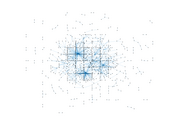### Degree distribution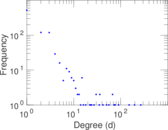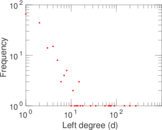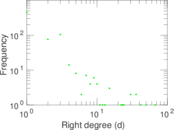### Cumulative degree distribution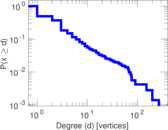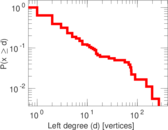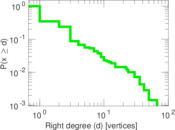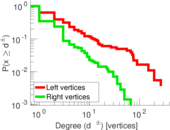### Lorenz curve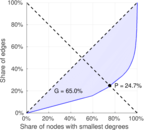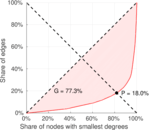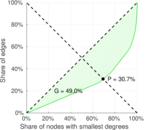### Spectral distribution of the adjacency matrix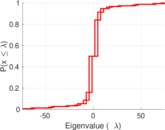### Spectral distribution of the normalized adjacency matrix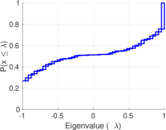### Spectral distribution of the Laplacian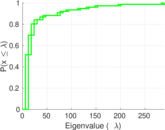### Spectral graph drawing based on the adjacency matrix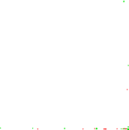### Spectral graph drawing based on the Laplacian### Spectral graph drawing based on the normalized adjacency matrix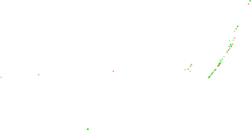### Degree assortativity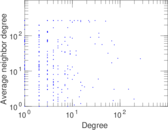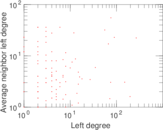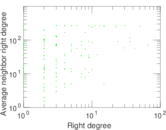### Zipf plot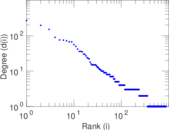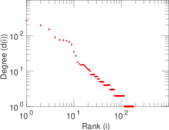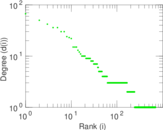### Hop distribution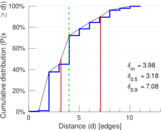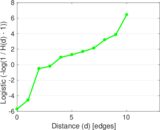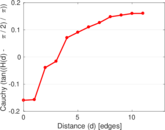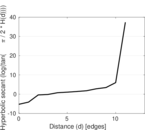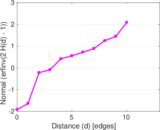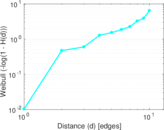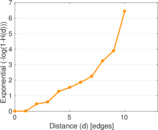### Double Laplacian graph drawing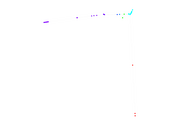### Delaunay graph drawing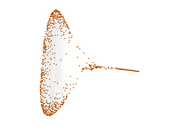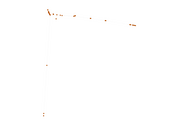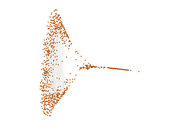### Edge weight/multiplicity distribution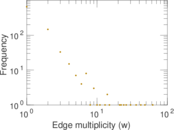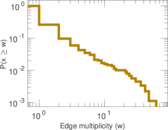### Temporal distribution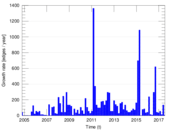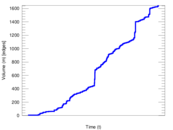### Temporal hop distribution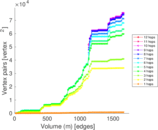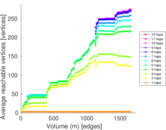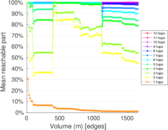### Diameter/density evolution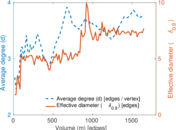### Matrix decompositions plots